# Arithmetic

Arithmetic

The teacher asked the class to find the value of $$3+7\times2$$

Mia and John were the first two to answer.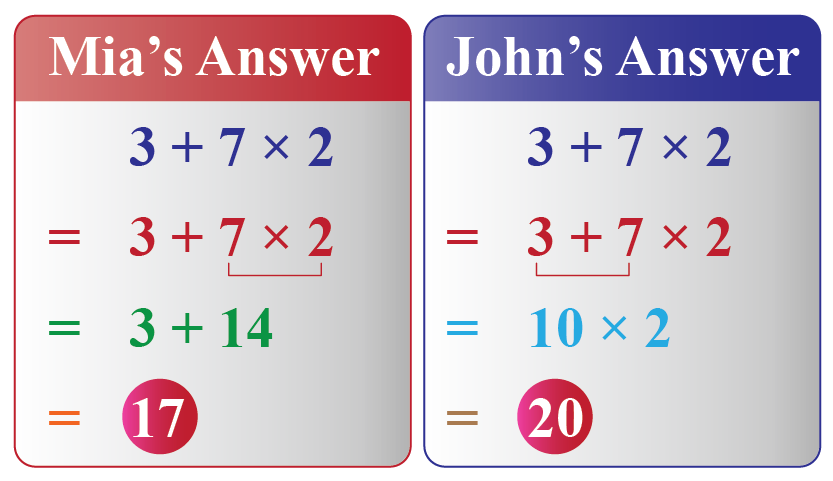Who do you think is correct?

It's Mia who got it right. Let's proceed to understand why her calculation is correct and what mistake John did. As we do so, we will explore the various concepts of arithmetic math and the operations involved.

## Lesson Plan

 1 What Is Arithmetic? 2 Important Notes on Arithmetic 3 Solved Examples on Arithmetic 4 Challenging Questions on Arithmetic 5 Interactive Questions on Arithmetic

## What Is Arithmetic?

Arithmetic is the branch of mathematics in which we study numbers and relations among numbers using various properties and use them to solve examples.

The word ‘Arithmetic’ comes from "arithmos", a Greek word meaning numbers. One of the oldest and fundamental principles of mathematics, Arithmetic is all about numbers and the elementary operations (addition, subtraction, multiplication, and division) that can be performed with those numbers.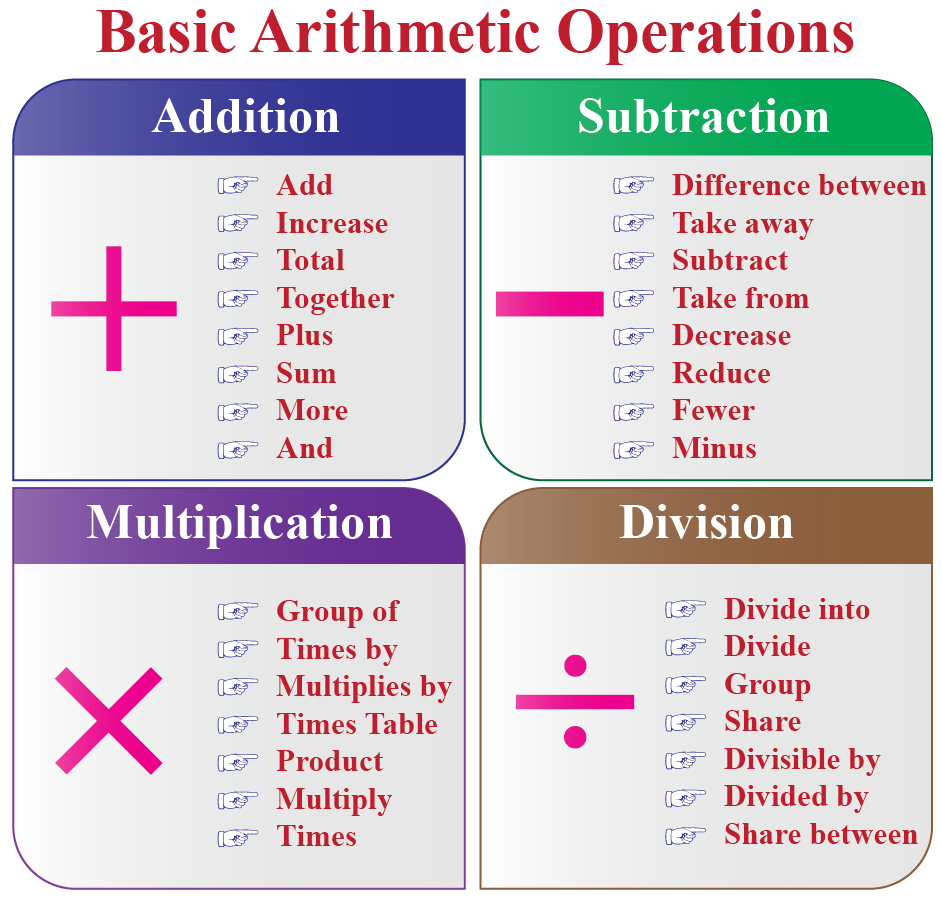Arithmetic is all around you. If you take out two ice cubes from the ice tray, how many are left? To find this, you will have to subtract 2 from the total number of slots.

If each room in your house has 3 windows, and there are 4 rooms, to find the total windows in the house you will have to multiply 3 with 4.

## Basic Rules of Arithmetic

Let's just briefly go over the basic arithmetics operations.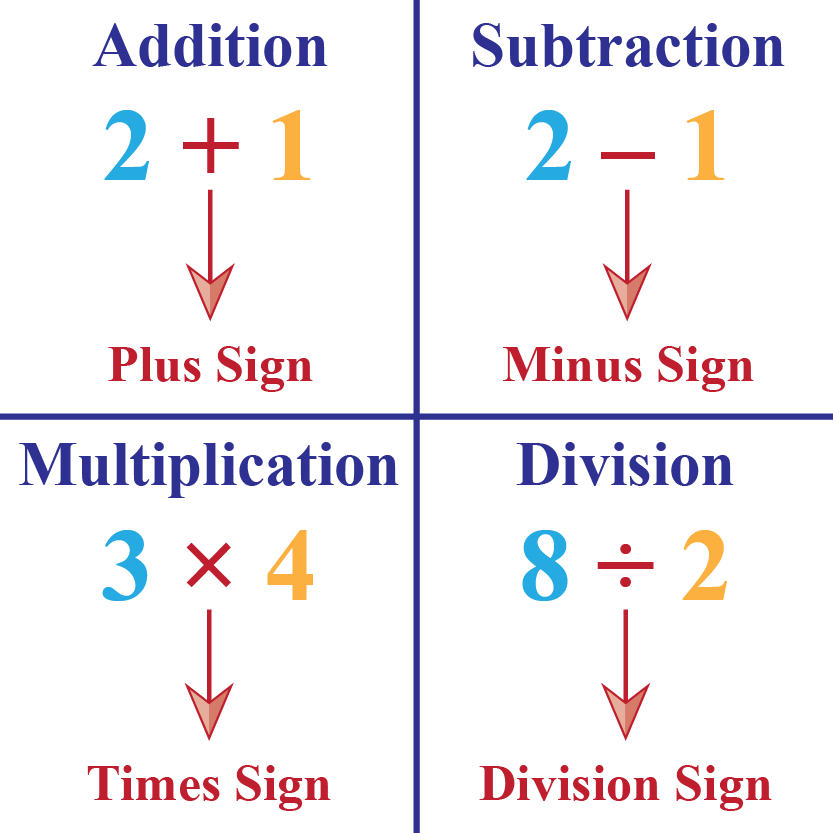Addition and subtraction are the most basic arithmetics operations. These concepts are building blocks of understanding and operating on numbers. We add and subtract numbers, amounts, and values in our everyday lives.

Addition can be visualized as 'putting together' of two or more quantities.

In arithmetics mathematics, subtraction means to take away some things from a group. In other words, subtraction is the process of removing things from a group.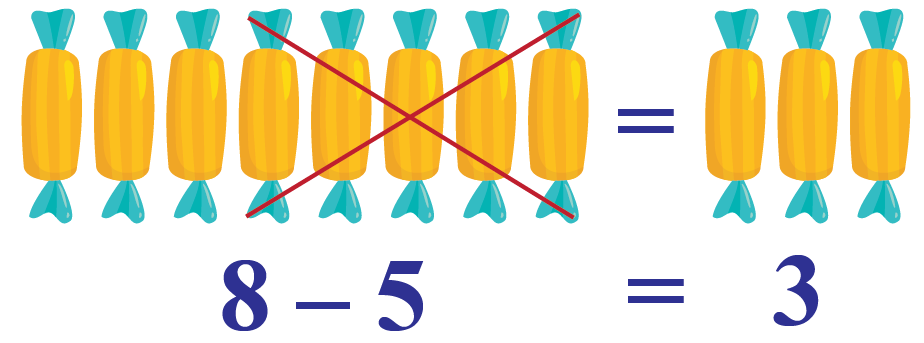### Multiplication and Division

Multiplication is one of the four basic arithmetic operations that can be applied to different math concepts like multiplying and dividing fractions, decimals, rationals, integers, etc. These operations form the building blocks for the other math concepts.

And the last of basic arithmetic operations is division. In simple words, the division can be defined as the splitting of a large group into equal smaller groups.

### Equal to Sign : "="

Equal to sign is used to denote the outcome of the operations on numbers.### Inverse Operations

• The operations addition and subtractions are inverse operators of each other.
• Similarly, the operations multiplication and division are inverse operators of each other.

### Example 1

When we add 3 to 8 we get 11. i.e. 8+3 = 11

This would also mean:

• If we take away 3 from 11, we get 8
• If we take away 8 from 11, we get 3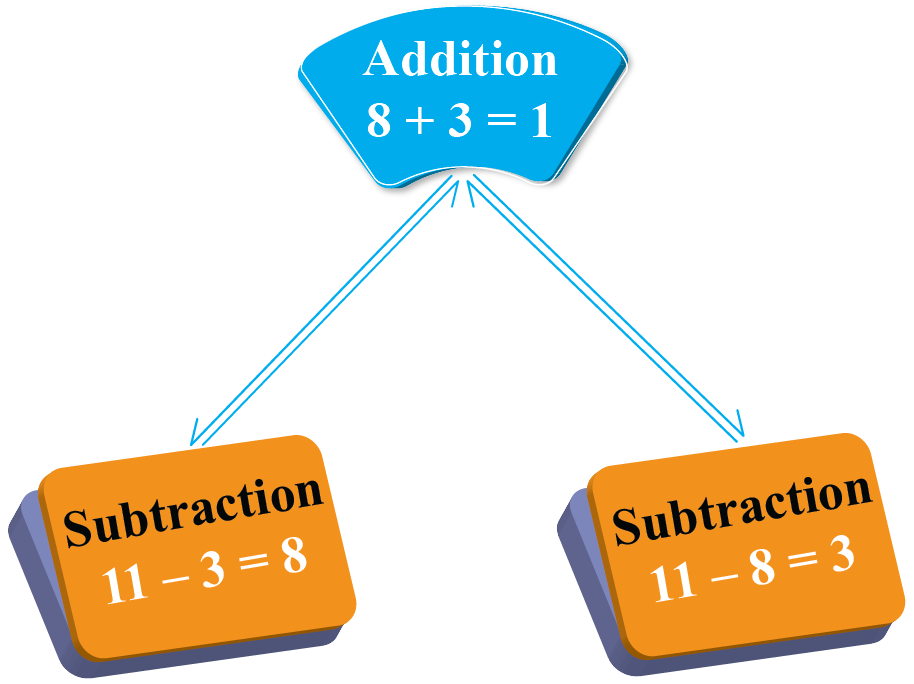### Example 2

Multiplying 3 with 8 gives 24 i.e 8 x 3 = 24

This would also mean:

• If we divide 24 by 3 we get 8
• If we divide 24 by 8 we get 3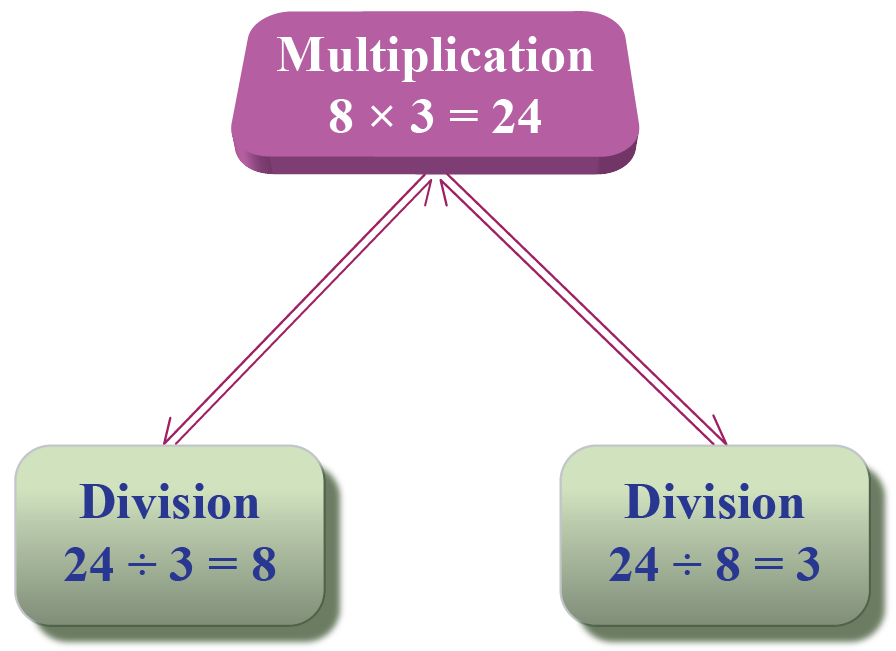When there is more than 1 operator present, there is a rule called DMAS which is to be followed to operate them.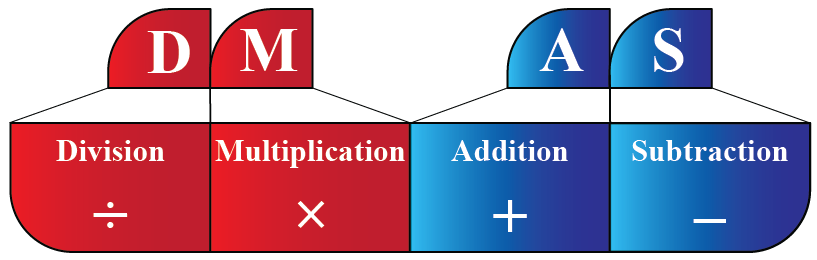As per this rule: When we operate numbers with multiple operators, going from left to right we need to first operate numbers involving division or multiplication followed by operators addition and subtraction.

Let's take the example that we took in the beginning, $$3+7\times2$$

Here, we have 2 operators, multiplication and addition.

So, the first multiplication of 7 and 2 will be done and then the answer will be added to 3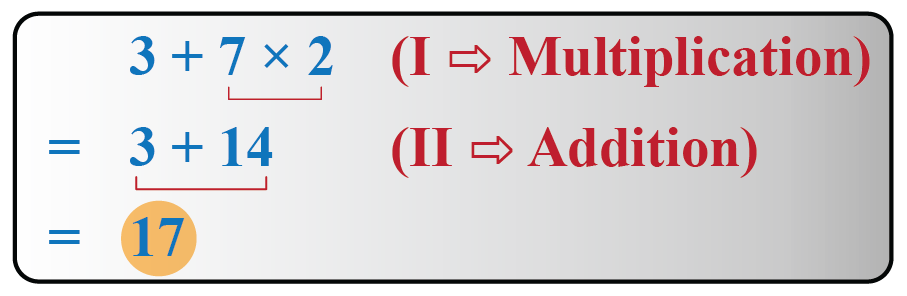## Arithmetic Examples

Let's quickly see some arithmetic examples from our day-to-day life.

### Example 1

Sia plucked 45 flowers from a garden and distributed them equally among 9 of her friends.

Can you find, how many flowers did each of her friends receive?

As we need to distribute 45 flowers equally among 9 children, we need to divide 45 by 9

$45\div 9 = 5$

So, each of her friends will receive 5 flowers.

### Example 2

Mother bought 4 packets of candies each for John and Mia. There were 5 candies inside each packet. Can you find total how many candies were there?

Number of candies in 1 packet = 5

So, number of candies in 4 packets = $$4\times5=20$$

Thus, each child will get 20 candies.

Number of children = 2

So, total number of candies = $$20\times2=40$$

More Important Topics
More Important Topics
Learn from the best math teachers and top your exams

• Live one on one classroom and doubt clearing
• Practice worksheets in and after class for conceptual clarity
• Personalized curriculum to keep up with school